# Java实现最小堆二

2016/07/04 19:01

Java实现最小堆二

n=0;
for(i=1;i<=m;i++)
{
n++;
h[n]=a[i];  //或者写成scanf("%d",&h[n]);
siftup();
}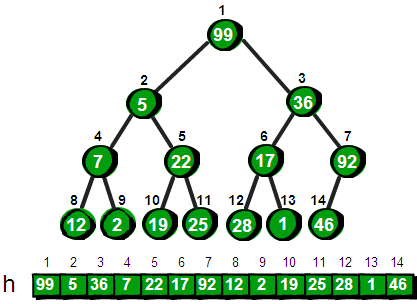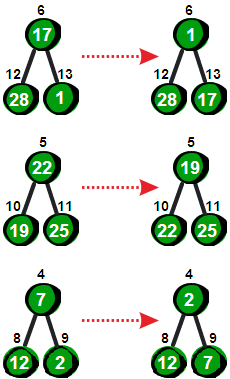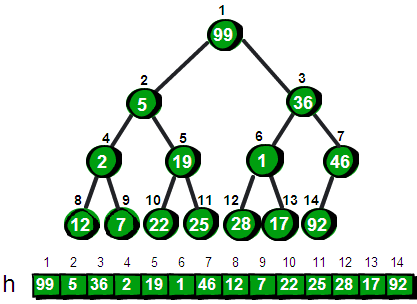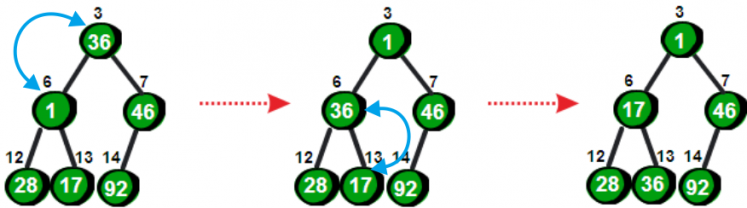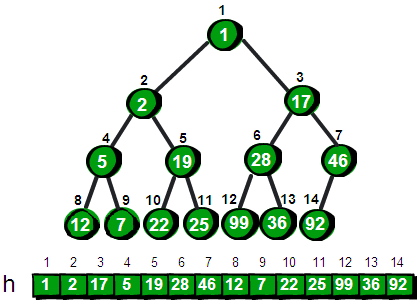for(i=n/2;i>=1;i--)
siftdown(i);

package com.usoft.heap;

import java.util.Random;

/**
* Created by xinxingegeya on 16/6/30.
*/
public class MinHeap<T extends Comparable<T>> {

/**
* 最小堆内部使用数组存储
*/
private Node<T>[] h;
private int maximumCapacity; //数组初始化大小
private int capacity; //堆的大小

private int index;

/**
* 最小堆的大小
*
* @param maxCapacity
*/
public MinHeap(int capacity, int maxCapacity) {
this.capacity = capacity;
this.maximumCapacity = maxCapacity;
h = (Node<T>[]) new Node[maxCapacity];
}

/**
* 使用Node封装最小堆的元素节点
*
* @param <T>
*/
static class Node<T extends Comparable<T>> implements Comparable<Node> {
private T data; //节点数据

public Node(T data) {
this.data = data;
}

public T getData() {
return data;
}

public void setData(T data) {
this.data = data;
}

@Override
public int compareTo(Node o) {
return this.data.compareTo((T) o.getData());
}
}

void swap(int x, int y) {
Node<T> t;
t = h[x];
h[x] = h[y];
h[y] = t;
}

//向下调整节点
void siftDown(int i) {//传入一个需要向下调整的结点编号i，这里传入1，即从堆的顶点开始向下调整
int t, flag = 0;//flag用来标记是否需要继续向下调整
//当i结点有儿子的时候（其实是至少有左儿子的情况下）并且有需要继续调整的时候循环窒执行
while (i * 2 <= capacity && flag == 0) {
//首先判断他和他左儿子的关系，并用t记录值较大的结点编号
if (h[i].compareTo(h[i * 2]) > 0) {
t = i * 2;
} else {
t = i;
}

//如果他有右儿子的情况下，再对右儿子进行讨论
if (i * 2 + 1 <= capacity) {
//如果右儿子的值更大，更新较小的结点编号
if (h[t].compareTo(h[i * 2 + 1]) > 0) {
t = i * 2 + 1;
}
}
//如果发现最大的结点编号不是自己，说明子结点中有比父结点更大的
if (t != i) {
swap(t, i);//交换它们，注意swap函数需要自己来写
i = t;//更新i为刚才与它交换的儿子结点的编号，便于接下来继续向下调整
} else {
flag = 1;//则否说明当前的父结点已经比两个子结点都要大了，不需要在进行调整了
}
}
}

//向上调整节点
void siftUp(int i) { //传入一个需要向上调整的结点编号i
int flag = 0; //用来标记是否需要继续向上调整
if (i == 1) return; //如果是堆顶，就返回，不需要调整了
//不在堆顶 并且 当前结点i的值比父结点小的时候继续向上调整
while (i != 1 && flag == 0) {
//判断是否比父结点的小
if (h[i].compareTo(h[i / 2]) < 0) {
swap(i, i / 2);//交换他和他爸爸的位置
} else {
flag = 1;//表示已经不需要调整了，当前结点的值比父结点的值要大
}
i = i / 2; //这句话很重要，更新编号i为它父结点的编号，从而便于下一次继续向上调整
}
}

/**
* 把数组初始化成最小堆
*/
void creatBySiftDown() {
int i;
//从最后一个非叶结点到第1个结点依次进行向上调整
for (i = capacity / 2; i >= 1; i--) {
siftDown(i);
}
}

/**
* 插入元素,并sift up
*
* @param data
*/
void insert(T data) {
if (capacity < maximumCapacity) {
Node<T> node = new Node<>(data);
h[++index] = node;
siftUp(index);
} else {
throw new RuntimeException("heap is full");
}
}

/**
* 使用最小堆的性质进行堆排序
*
* @return
*/
Node deleteMin() {
Node node;
node = h;
h = h[capacity];
capacity--;
siftDown(1);
return node;
}

public static void main(String args[]) {

int maxCapacity = 100;
int capacity = 9;
MinHeap<Item> minHeap = new MinHeap<>(capacity, maxCapacity);

for (int i = 0; i < capacity; i++) {
Random random = new Random();
int r = random.nextInt();
Item item = new Item(Math.abs(r) % 1000);
minHeap.insert(item);
}

for (int i = 1; i <= capacity; i++) {
System.out.print(minHeap.h[i].getData().getId() + ",");
}

System.out.println();

for (int i = 1; i <= capacity; i++) {
System.out.println(((Item) minHeap.deleteMin().getData()).getId());
}
}
}



========END========

1
3 收藏

### 作者的其它热门文章0 评论
3 收藏
1